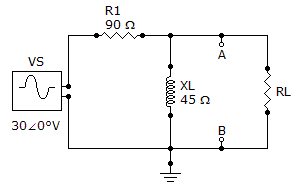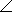# Electrical Engineering - Circuit Theorems in AC Analysis - Discussion

### Discussion :: Circuit Theorems in AC Analysis - General Questions (Q.No.1)

1.

Determine VTH when R1 is 180and XL is 90.[A]. 13563.4° V [B]. 13.563.4° V [C]. 12.20° V [D]. 1220° V

Explanation:

No answer description available for this question.

 Anand M said: (Dec 15, 2011) I want no the explanation for this quation.

 Alfredo said: (Mar 18, 2012) Why is XL given in ohms? Shouldn't it be henries?

 James said: (Apr 24, 2012) Xl also has some form of resistance

 Cherry said: (May 9, 2012) Simply replace with 180 and 90 and solve 1st find out the current in the loop ...to find Vth 1. 30/sqrtof(180 and 90) with angle ll get 2. then multiply with Xl to get Vth 0.15*90 at angle 90 Ans.13.25 at an angle 63.4

 Gary said: (Aug 16, 2012) Can't be (A), load voltage is higher than the source. Can't be (C) or (D), as the phase angle is zero. Even without doing the calculations, it has to be (B).

 Vijaya said: (Mar 28, 2013) Give simple technique for this type of problems.

 Jai said: (Sep 26, 2013) voltage across A-B = Voltage across XL = Vth. By volt div. rule, Vth = (30*j90)/(180+j90). = 13.5 ang(63.4).

 Nikhil Jain said: (Jun 29, 2014) The value of inductance should be given in the form of j or in henry.

 Nikhil Jain said: (Oct 9, 2014) Can't be (A) , load voltage is higher than the source. Can't be (C) or (D) , as the phase angle is zero. Even without doing the calculations, it has to be (B).

 Vasiq said: (Jan 18, 2015) @Jai. That is not correct. 90 is the value of the resistor and, hence, shouldn't have a joint in front of it.

 Dhananjay said: (Jun 11, 2015) This can be solved simply. First find total impedance "Z" then current in the branch. Then multiply with the inductor reactance that is X. z = sqrt of (R^2 + X^2) where R = resistance = 180 and X = reactant = 90. Solve it. Find i = supply voltage/impedance (Z) = 30/calculated z. Now to get Vth multiply calculated current with reactance (x=90). Then to find phase angle we know that tan Q = x/r.

 Jai said: (Jan 14, 2016) Explain phase angle?

 Ravinder said: (Jul 13, 2016) By using volt division rule Vab = Vs * XL/ (R1 + XL) which give Vab = 10V.

 Bruce said: (Aug 3, 2016) In a 3 phase cct, 380v, 1 breaker per phase. Q: when all breakers powered, they all trip, why?

 Hamimullah said: (Nov 1, 2018) How to find the phase angle? Anyone explain me.

 Riya said: (Apr 28, 2019) Actually, l is inductance but xl is the impedance of that device. Impedance is simply resistance. Hence it is in ohms.